top of page
Search
• Ekta Aggarwal

# Data Structures in Python

To handle and store the data in an efficient manner python has various data structures - each having its own utility. Python has 4 types of data structures which we shall be understanding in detail:

## Lists

Lists are a data object in Python which can store multiple values and the elements need not be of same data type. A list is created in [ ] square brackets.

1. A list can have multiple elements

2. Elements of different data types: Following is our list of scores - which contains student names (strings) and their scores (numeric)

`Scores = ['Emma',78,'Rebecca',80,'John',98,'Thomas',41,'Robin',66]`

3. A list can contain several lists! Following list contains scores of students where each element is itself a list!

`list2 = [['Emma',78],['Rebecca',80],['John',98],['Thomas',41],['Robin',66]]`

### Subsetting lists

Understanding with the help of an example!

`Scores = ['Emma',78,'Rebecca',80,'John',98,'Thomas',41,'Robin',66]`

Python indexing begins from 0. Thus to get the first element of the list we write:

list_name . Square brackets denote that we want to extract an element from our list.

`Scores`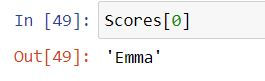Following code will return fourth element (indexing starts from 0, thus 3 represents 4th index)

`Scores`Writing a semi-colon : in square brackets [ ] in front of the list name would return all the elements.

`Scores[:]`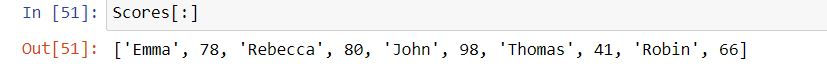In Python an index of -1 indicates extract the last element of our list!

`Scores[-1]`## List Slicing

Extracting multiple elements of a list is called list slicing (you are slicing down i.e. cutting down your list)

`Scores[1:4] # Extract the elements from first index to third index (index 4 is excluded) i.e. positions 2 to 4. (Remember Python indexing starts from 0)`If we write [N: ] in front of a list then it tells Python to extract all the elements starting from Nth index.

`Scores[1:]`If we write [:N] in front of a list then it tells Python to extract elements from the starting till the (N-1)th index (N is excluded!)

`Scores[:4]`### Changing the elements

By defining list_name[index] = 'New_value' we can update the values in our list

```Scores = 'Asif'
Scores```We can also change multiple elements of a list in one command. In the following code we are updating 0th and 1st index values as 'Lisa' and 86

```Scores[0:2] = ['Lisa',86]
Scores```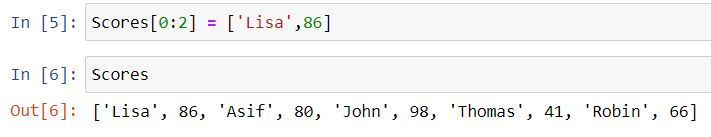We can also append or add elements in our list.

Method 1: Using a + symbol we can add elements to our list.

In the following code we are adding a list ['Henry',92] at the end of our original list Scores.

```Scores = Scores + ['Henry',92];
print(Scores)```Method 2: Using .append( ) command

In the following code we are adding an element "Bella" at the end of our list Scores.

```Scores.append('Bella');
print(Scores)```### Removing elements from a list

We can remove elements from a list using .remove( ) command:

```Scores.remove('Bella');
print(Scores)```We can also insert elements by specifying the index using insert ( ) function. In the following code we have added Bella at 2nd index (i.e. 3rd position) in our list.

```Scores.insert(2,'Bella');
print(Scores)```Let us remove this element for the sake of simplicity.

```Scores.remove('Bella');
print(Scores)```

We can also remove the elements by specifying the index using pop( ) command. In the code below we are deleting the element at 3rd index i.e. 4th position.

```Scores.pop(3);
print(Scores)```If you do not specify anything in the pop( ) command then by default it removes the last element from the list.

```Scores.pop();
print(Scores)```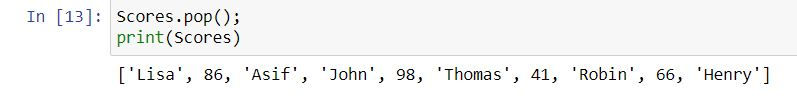## Tuples

Unlike lists tuples are the data structures where the elements cannot be altered. A tuple is created using parenthesis ( )

```Scores = (78,80,98,41,66);
type(Scores)```### Subsetting a tuple

A tuple can be filtered like a list. Its indexing starts from 0.

```Scores = ('Emma',78,'Rebecca',80,'John',98,'Thomas',41,'Robin',66)
Scores
Scores```You cannot alter or change the values in a tuple after it is created. For example in the following code we are trying to replace the value Emma by Henry. If it would have been a list then we could have made this change successfully. But alas! Tuples don't allow us to change their values.

`Scores['Emma'] = 'Henry'`### Assigning values of tuples to multiple variables

Let us create a tuple

`Scores = (78,80,98,41,66)`

We can assign these scores individually to each of the variables as follows:

```Emma,Rebecca, John,Thomas, Robin = Scores
Emma```

Here Emma gets the value 78, Rebecca as 80, John as 98 etc.To learn about lists in detail refer to this tutorial!

## Dictionaries

Python has a special object dictionaries which store the mapping in form of key and values, where value(s) map to a single key. In this tutorial we shall learn about dictionaries in depth.

Keys in dictionaries must be unique and are case -sensitive i.e. X and x will be treated as 2 different keys.

Dictionaries are denoted by { } curly brackets.

Let us create our first dictionary with key value pairs denoting Countries and their capitals.

The mapping between keys and values are denoted by : colon .

Different key-value pairs are separated by a comma.

```my_dict = {'U.S.A' : 'Washington D.C',
'U.K.' : 'London',
'Japan':'Tokyo',
'Russia':'Moscow'}

type(my_dict)```### Items

Items in a python dictionary are the key-value pairs. In the above dictionary we have 4 items. Items can be retrieved by:

`my_dict.items()`### Keys

Keys in dictionaries are unique and are used as one to many or one to one mapping for values. In our dictionary we have defined countries as our keys.

`my_dict.keys()`### Values

Values in a dictionary can be a one-dimensional list or single values of any variable type (numeric or string or boolean). They can be repetitive and get mapped to keys.

In my_dict we have defined capitals as the values.

`my_dict.values()`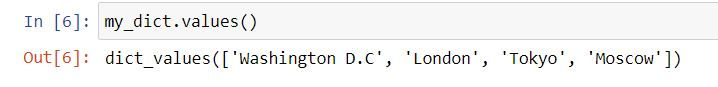### Adding an item to our dictionary

We can add a new item (key-value pair) in following syntax:

dict_name['key'] = values.

Task: Add a new key - value pair 'India : New Delhi' to our dictionary my_dict.

```my_dict['India'] = 'New Delhi'
my_dict```### Deleting an item from our dictionary

We can delete a key value pair from our dictionary using del keyword with following syntax:

del dictionary_name['key_to_be_deleted']

Task: Delete key 'India' from our dictionary.

```del my_dict['India']
my_dict```### Mapping multiple values to a single key.

In a dictionary we can map multiple values to a key. The multiple values are passed in the form of a list!

Let us create a dictionary with 2 keys: Countries and Capitals, where they values will be passed on the form of lists.

```my_dict = {'Countries' : ['U.S.A.','U.K.','Japan','Russia'],
'Capitals' : ['Washington D.C.','London','Tokyo','Moscow']}
my_dict``````my_dict.items()
my_dict.keys()
my_dict.values()```

## Sets

Sets are immutable objects in python which are created using { } curly brackets. They always have unique values.

In the following set, we are repeating Japan twice but a set removes the duplicates.

```my_set = {'U.S.A.','U.K.','Russia','Japan','Japan'}
my_set```### Adding a value to the set

We can add the values in a set using add( ) command.

```my_set.add('India')
my_set```### Removing the values from a set

Values in a set can be removed using remove( ) function.

```my_set.remove('India')
my_set```bottom of page